Type
Quiz
Book Title
Fundamentals of Corporate Finance Standard Edition 9th Edition
ISBN 13
978-0073382395

### 978-0073382395 Chapter 17 Questions and Problems

April 3, 2019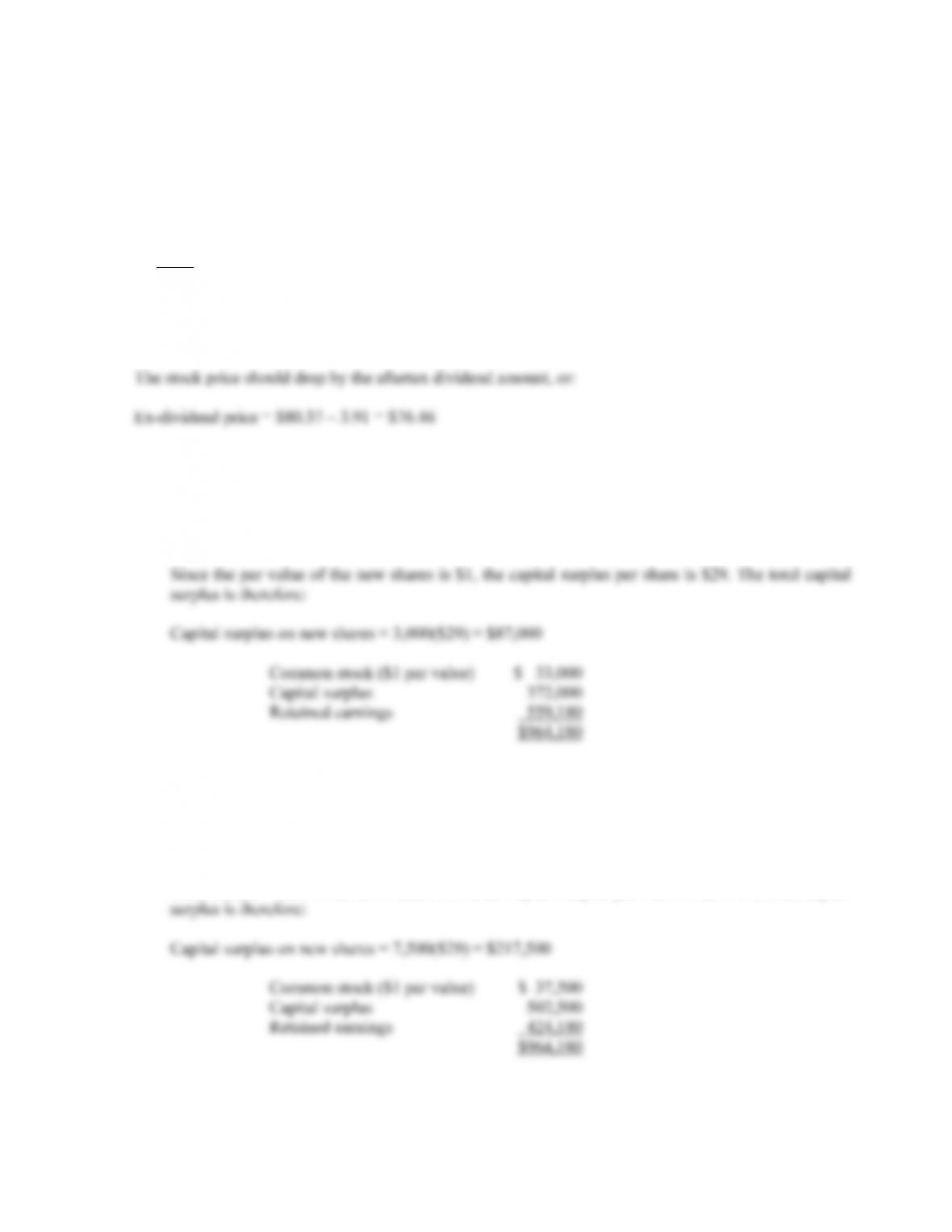CHAPTER 17 B-293
Solutions to Questions and Problems
NOTE: All end of chapter problems were solved using a spreadsheet. Many problems require multiple steps.
Due to space and readability constraints, when these intermediate steps are included in this solutions
manual, rounding may appear to have occurred. However, the final answer for each problem is found
without rounding during any step in the problem.
Basic
1. The aftertax dividend is the pretax dividend times one minus the tax rate, so:
Aftertax dividend = \$4.60(1 – .15) = \$3.91
2. a. The shares outstanding increases by 10 percent, so:
New shares outstanding = 30,000(1.10) = 33,000
New shares issued = 3,000
b. The shares outstanding increases by 25 percent, so:
New shares outstanding = 30,000(1.25) = 37,500
New shares issued = 7,500
Since the par value of the new shares is \$1, the capital surplus per share is \$29. The total capital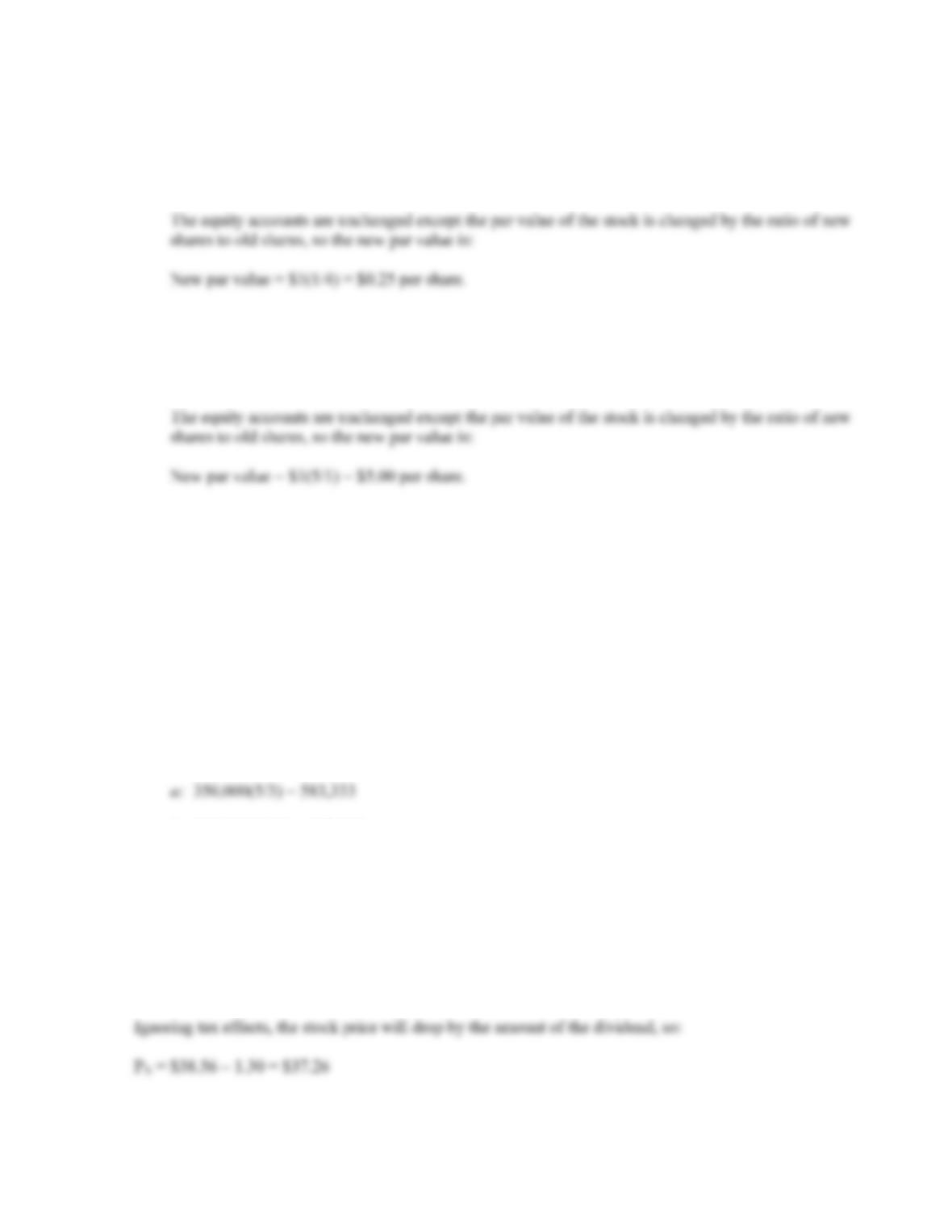B-294 SOLUTIONS
3. a. To find the new shares outstanding, we multiply the current shares outstanding times the ratio of
new shares to old shares, so:
New shares outstanding = 30,000(4/1) = 120,000
b. To find the new shares outstanding, we multiply the current shares outstanding times the ratio of
new shares to old shares, so:
New shares outstanding = 30,000(1/5) = 6,000.
4. To find the new stock price, we multiply the current stock price by the ratio of old shares to new shares,
so:
a. \$90(3/5) = \$54.00
b. \$90(1/1.15) = \$78.26
c. \$90(1/1.425) = \$63.16
d. \$90(7/4) = \$157.50
e. To find the new shares outstanding, we multiply the current shares outstanding times the ratio of
new shares to old shares, so:
b: 350,000(1.15) = 402,500
c: 350,000(1.425) = 498,750
d: 350,000(4/7) = 200,000
5. The stock price is the total market value of equity divided by the shares outstanding, so:
P
0 = \$308,500 equity/8,000 shares = \$38.56 per share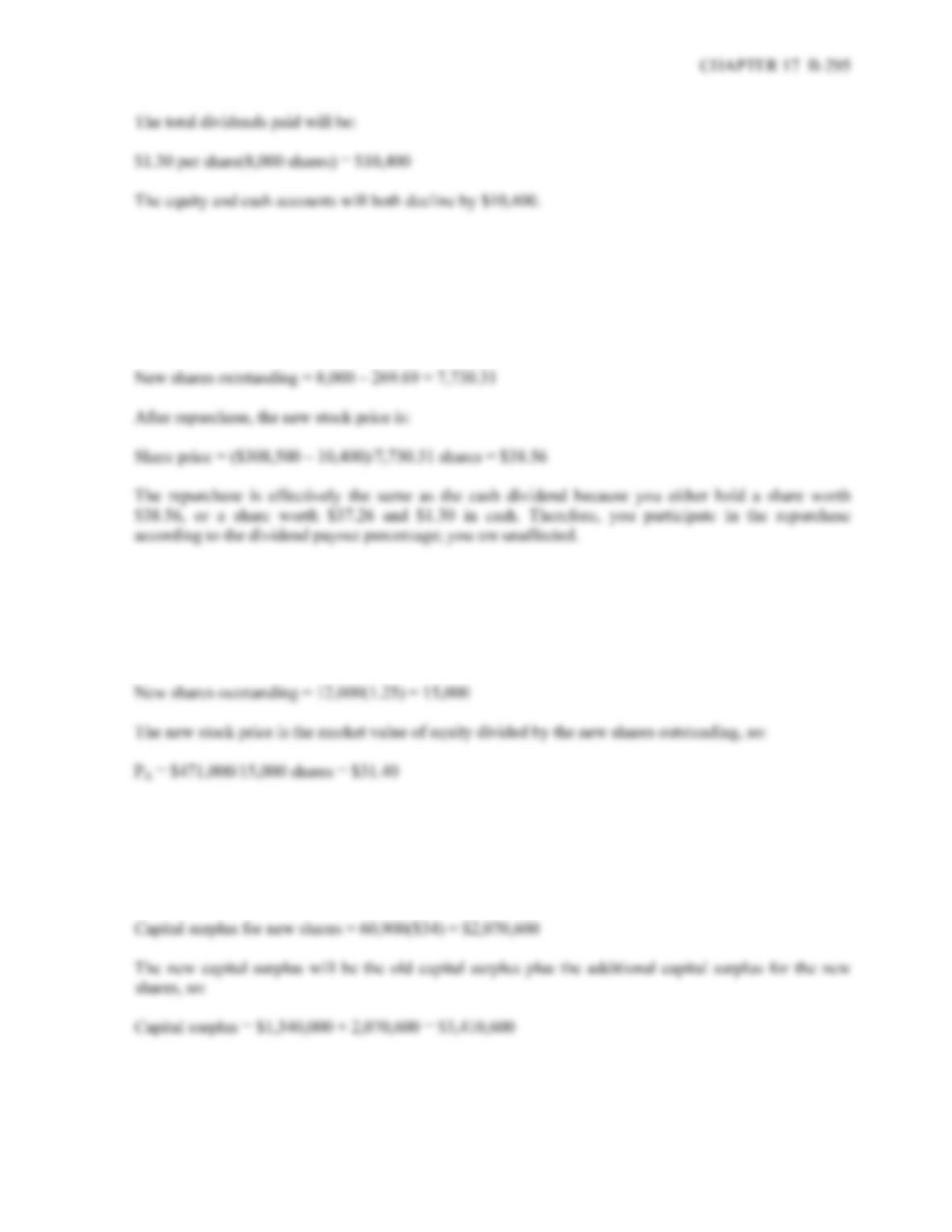6. Repurchasing the shares will reduce cash and shareholders’ equity by \$10,400. The shares repurchased
will be the total purchase amount divided by the stock price, so:
Shares bought = \$10,400/\$38.56 = 269.69
And the new shares outstanding will be:
7. The stock price is the total market value of equity divided by the shares outstanding, so:
P
0 = \$471,000 equity/12,000 shares = \$39.25 per share
The shares outstanding will increase by 25 percent, so:
8. With a stock dividend, the shares outstanding will increase by one plus the dividend amount, so:
New shares outstanding = 406,000(1.15) = 466,900
The capital surplus is the capital paid in excess of par value, which is \$1, so: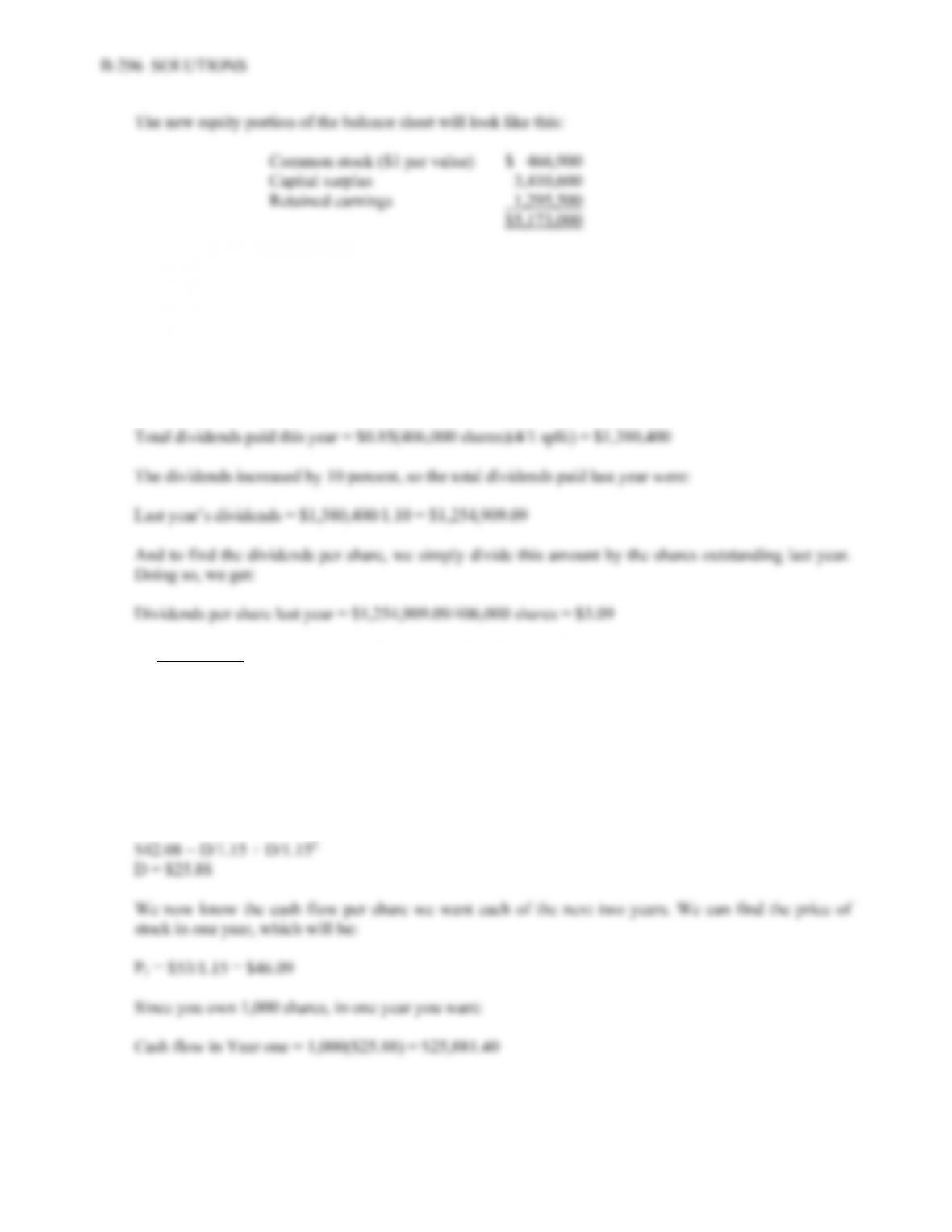9. The only equity account that will be affected is the par value of the stock. The par value will change by
the ratio of old shares to new shares, so:
New par value = \$1(1/4) = \$0.25 per share.
The total dividends paid this year will be the dividend amount times the number of shares outstanding.
The company had 406,000 shares outstanding before the split. We must remember to adjust the shares
outstanding for the stock split, so:
Intermediate
10. The price of the stock today is the PV of the dividends, so:
P
0 = \$2.30/1.15 + \$53/1.152 = \$42.08
To find the equal two year dividends with the same present value as the price of the stock, we set up the
following equation and solve for the dividend (Note: The dividend is a two year annuity, so we could
solve with the annuity factor as well):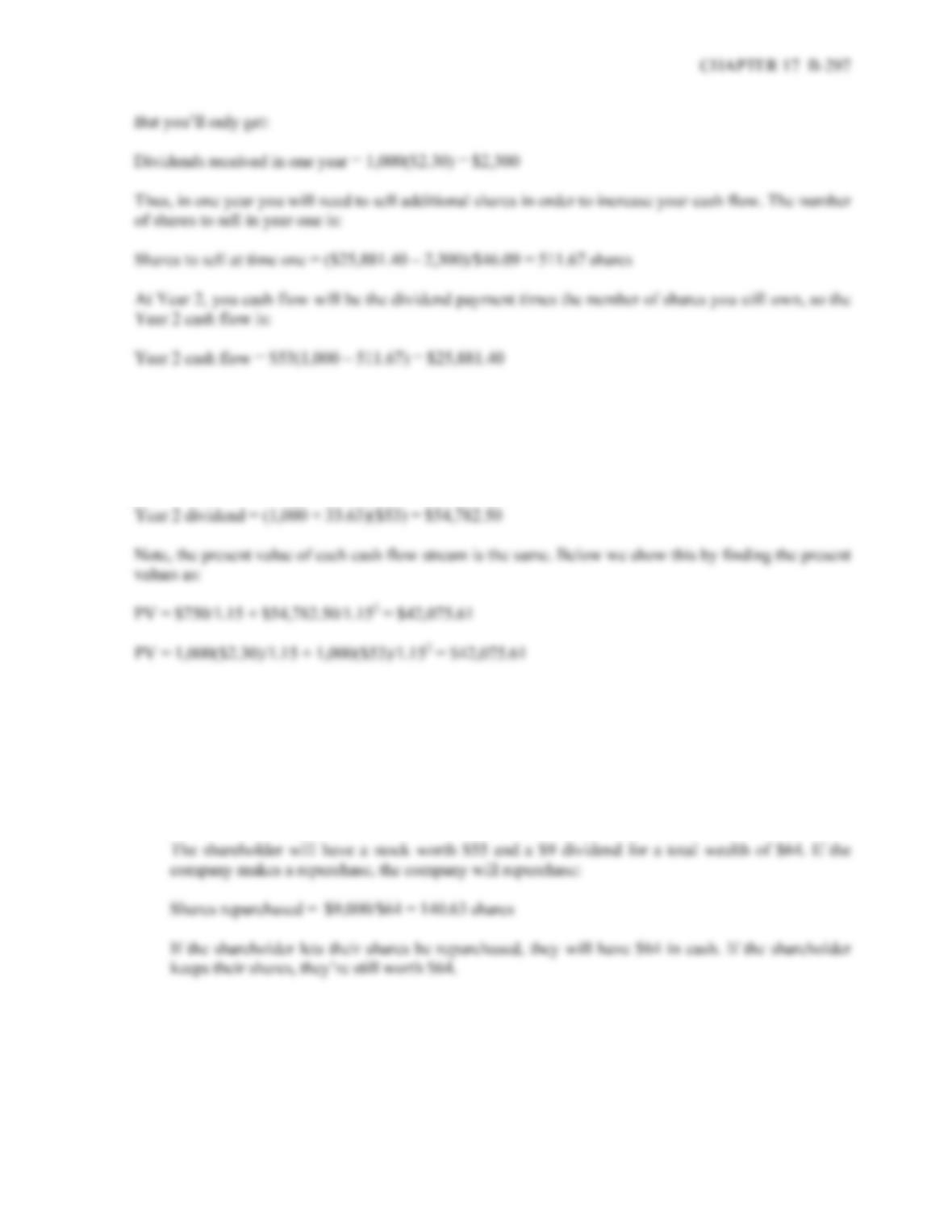11. If you only want \$750 in Year 1, you will buy:
(\$2,300 – 750)/\$46.09 = 33.63 shares
at time 1. Your dividend payment in Year 2 will be:
12. a. If the company makes a dividend payment, we can calculate the wealth of a shareholder as:
Dividend per share = \$9,000/1,000 shares = \$9.00
The stock price after the dividend payment will be:
P
X = \$64 – 9 = \$55 per share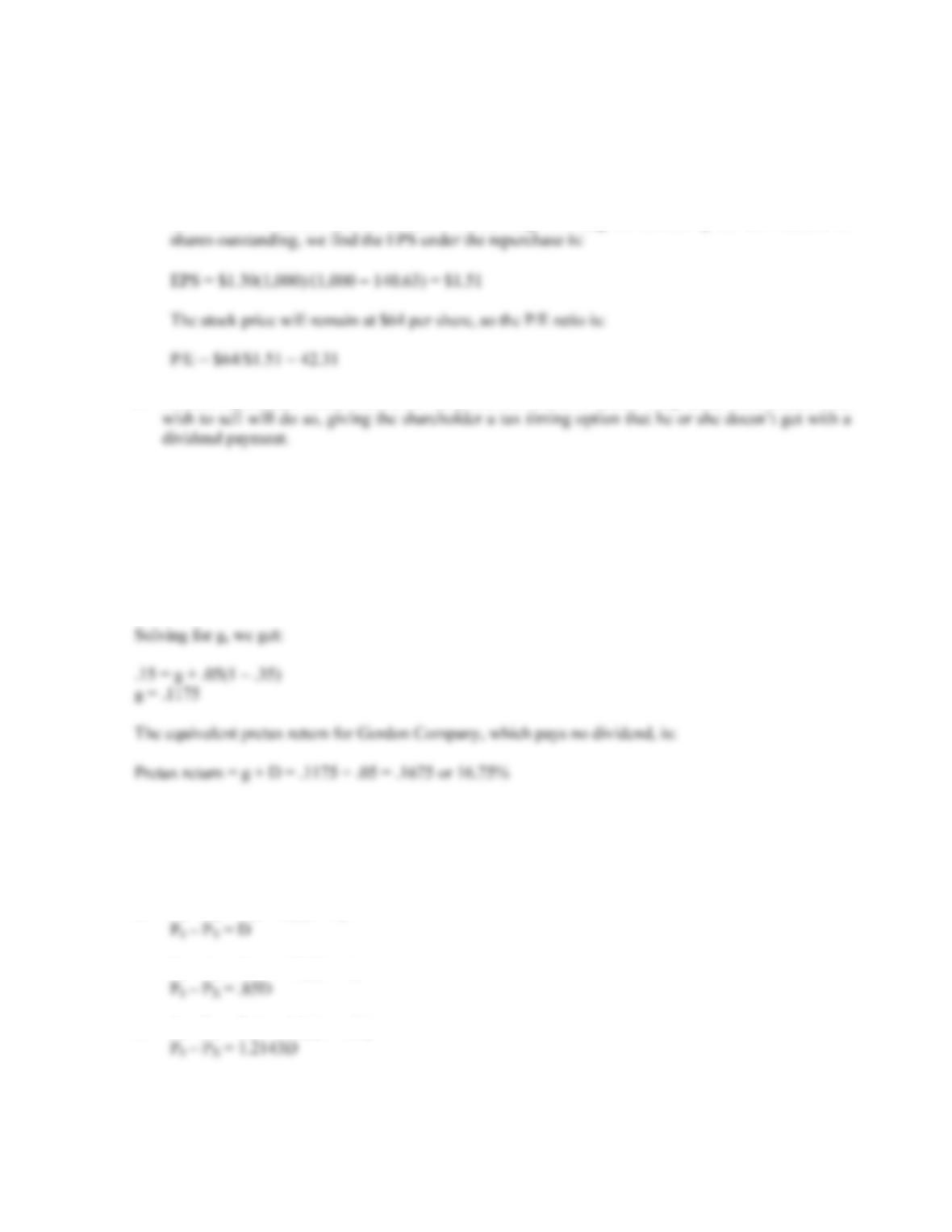B-298 SOLUTIONS
b. If the company pays dividends, the current EPS is \$1.30, and the P/E ratio is:
P/E = \$55/\$1.30 = 42.31
If the company repurchases stock, the number of shares will decrease. The total net income is the
EPS times the current number of shares outstanding. Dividing net income by the new number of
c. A share repurchase would seem to be the preferred course of action. Only those shareholders who
Challenge
13. Assuming no capital gains tax, the aftertax return for the Gordon Company is the capital gains growth
rate, plus the dividend yield times one minus the tax rate. Using the constant growth dividend model,
we get:
Aftertax return = g + D(1 – t) = .15
14. Using the equation for the decline in the stock price ex-dividend for each of the tax rate policies,
we get:
(P0 – PX)/D = (1 – TP)/(1 – TG)
a. P
0 – PX = D(1 – 0)/(1 – 0)
b. P
0 – PX = D(1 – .15)/(1 – 0)
c. P
0 – PX = D(1 – .15)/(1 – .30)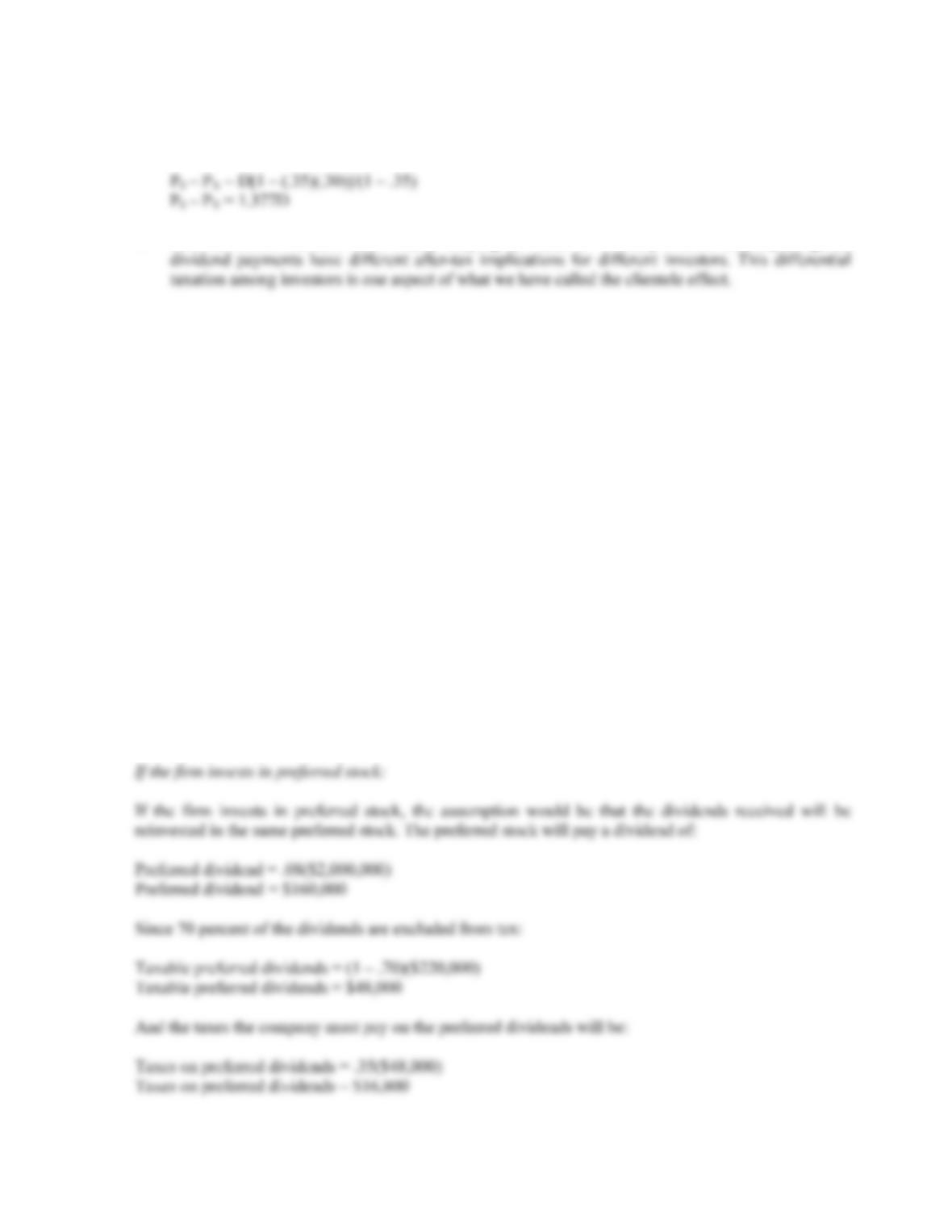CHAPTER 17 B-299
d. With this tax policy, we simply need to multiply the personal tax rate times one minus the dividend
exemption percentage, so:
e. Since different investors have widely varying tax rates on ordinary income and capital gains,
15. Since the \$2,000,000 cash is after corporate tax, the full amount will be invested. So, the value of each
alternative is:
Alternative 1:
The firm invests in T-bills or in preferred stock, and then pays out as special dividend in 3 years
If the firm invests in T-Bills:
If the firm invests in T-bills, the aftertax yield of the T-bills will be:
Aftertax corporate yield = .05(1 – .35)
Aftertax corporate yield = .0325 or 3.25%
So, the future value of the corporate investment in T-bills will be:
FV of investment in T-bills = \$2,000,000(1 + .0325)3
FV of investment in T-bills = \$2,201,406.16
Since the future value will be paid to shareholders as a dividend, the aftertax cash flow will be:
Aftertax cash flow to shareholders = \$2,201,406.16(1 – .15)
Aftertax cash flow to shareholders = \$1,871,195.23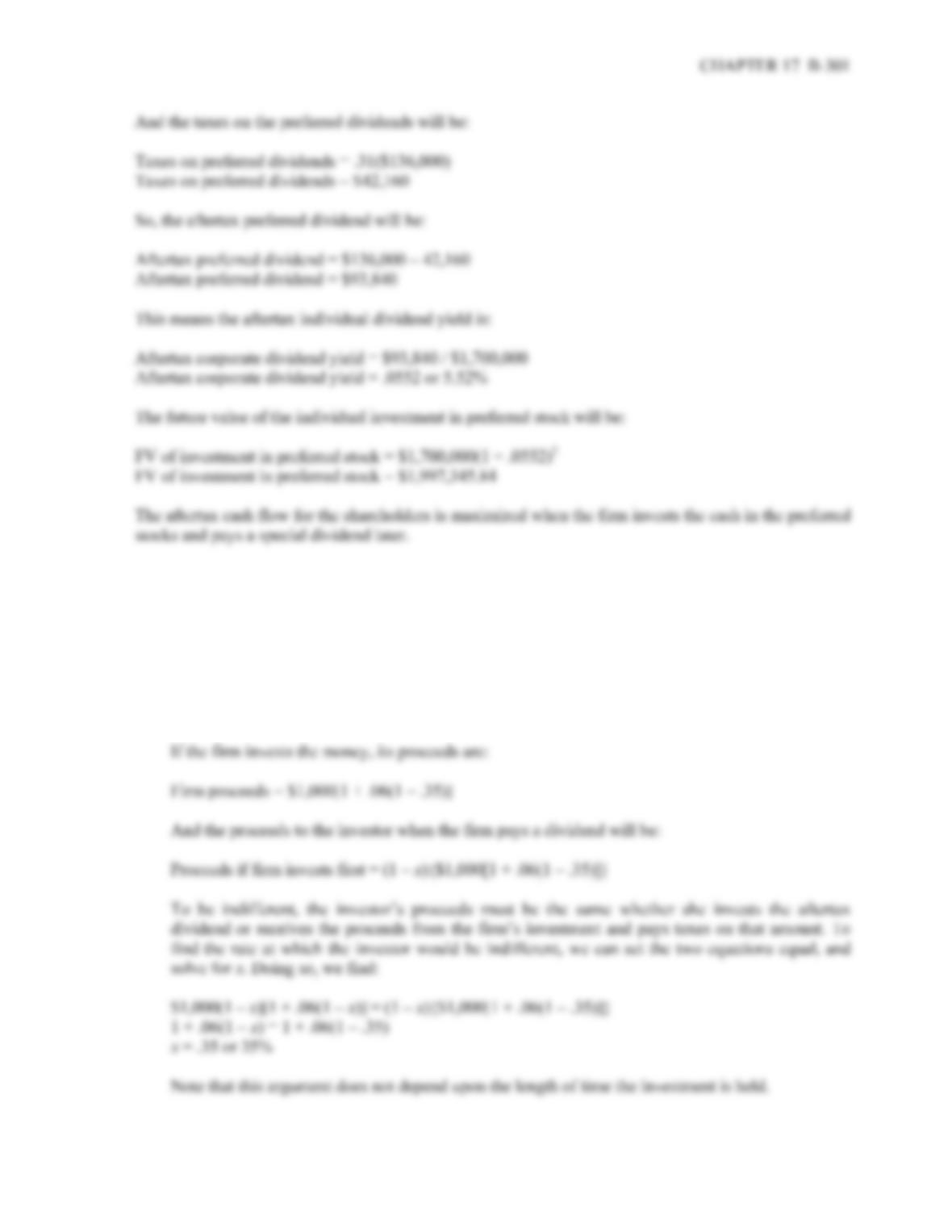16. a. Let x be the ordinary income tax rate. The individual receives an after-tax dividend of:
Aftertax dividend = \$1,000(1 – x)
which she invests in Treasury bonds. The Treasury bond will generate aftertax cash flows to the
investor of:
Aftertax cash flow from Treasury bonds = \$1,000(1 – x)[1 + .06(1 – x)]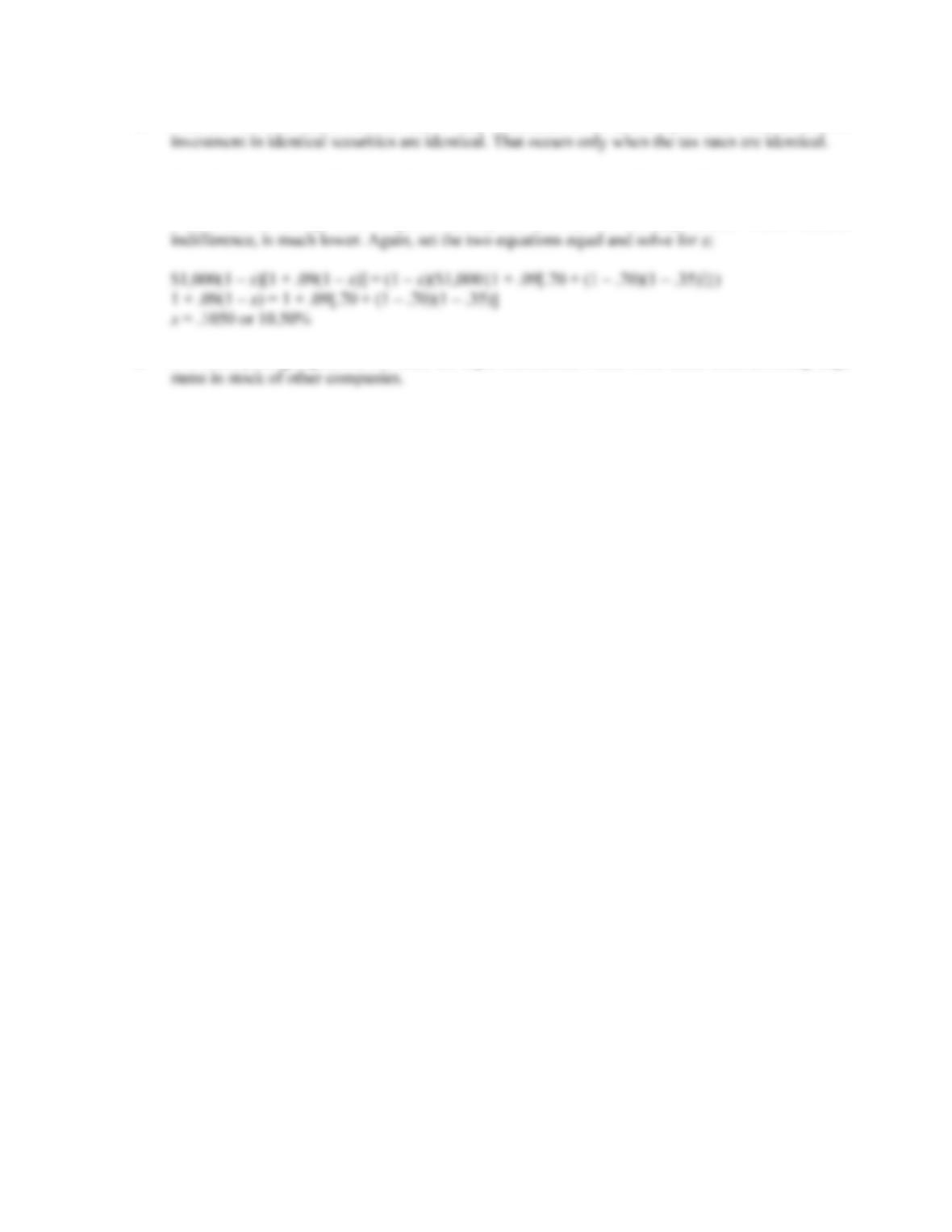B-302 SOLUTIONS
b. Yes, this is a reasonable answer. She is only indifferent if the aftertax proceeds from the \$1,000
c. Since both investors will receive the same pre-tax return, you would expect the same answer as in
part a. Yet, because Carlson enjoys a tax benefit from investing in stock (70 percent of income
from stock is exempt from corporate taxes), the tax rate on ordinary income which induces
d. It is a compelling argument, but there are legal constraints, which deter firms from investing large